# Data reclassification¶

Reclassifying data based on specific criteria is a common task when doing GIS analysis. The purpose of this lesson is to see how we can reclassify values based on some criteria which can be whatever, such as:

1. if available space in a pub is less than the space in my wardrobe

AND

2. the temperature outside is warmer than my beer

------------------------------------------------------

IF TRUE: ==> I go and drink my beer outside
IF NOT TRUE: ==> I go and enjoy my beer inside at a table


Even though, the above would be an interesting study case, we will use slightly more traditional cases to learn classifications. We will use Corine land cover layer from year 2012, and a Travel Time Matrix data from Helsinki to classify some features of them based on our own self-made classifier, or using a ready made classifiers that are commonly used e.g. when doing visualizations.

The target in this part of the lesson is to:

1. classify the lakes into big and small lakes where

• a big lake is a lake that is larger than the average size of all lakes in our study region
• a small lake ^ vice versa
2. use travel times and distances to find out

• good locations to buy an apartment with good public tranportation accessibility to city center
• but from a bit further away from city center where the prices are lower (or at least we assume so).
3. use ready made classifiers from pysal -module to classify travel times into multiple classes.

You should have following Shapefiles in the data folder:

$cd /home/geo/L4/data$ ls
Corine2012_Uusimaa.cpg      Helsinki_borders.cpg                       TravelTimes_to_5975375_RailwayStation.dbf
Corine2012_Uusimaa.dbf      Helsinki_borders.dbf                       TravelTimes_to_5975375_RailwayStation.prj
Corine2012_Uusimaa.prj      Helsinki_borders.prj                       TravelTimes_to_5975375_RailwayStation.shp
Corine2012_Uusimaa.shp      Helsinki_borders.shp                       TravelTimes_to_5975375_RailwayStation.shx
Corine2012_Uusimaa.shp.xml  Helsinki_borders.shx
Corine2012_Uusimaa.shx      TravelTimes_to_5975375_RailwayStation.cpg


## Data preparation¶

Before doing any classification, we need to prepare our data a little bit.

Let’s read the data in and select only English columns from it and plot our data so that we can see how it looks like on a map.

import geopandas as gpd
import matplotlib.pyplot as plt

# File path
fp = "/home/data/Corine2012_Uusimaa.shp"



Let’s see what we have.

In : data.head(2)
Out:
Level1            Level1Eng          Level1Suo  Level2     Level2Eng  \
0       1  Artificial surfaces  Rakennetut alueet      11  Urban fabric
1       1  Artificial surfaces  Rakennetut alueet      11  Urban fabric

Level2Suo  Level3                   Level3Eng  \
0  Asuinalueet     112  Discontinuous urban fabric
1  Asuinalueet     112  Discontinuous urban fabric

Level3Suo Luokka3  \
0  Väljästi rakennetut asuinalueet     112
1  Väljästi rakennetut asuinalueet     112

geometry
0  POLYGON ((279500 6640640, 279507.469 6640635.3...
1  POLYGON ((313620 6655820, 313639.8910000001 66...


Let’s select only English columns

# Select only English columns
In : selected_cols = ['Level1', 'Level1Eng', 'Level2', 'Level2Eng', 'Level3', 'Level3Eng', 'Luokka3', 'geometry']

# Select data
In : data = data[selected_cols]

# What are the columns now?
In : data.columns
Out:
Index(['Level1', 'Level1Eng', 'Level2', 'Level2Eng', 'Level3', 'Level3Eng',
'Luokka3', 'geometry'],
dtype='object')


Let’s plot the data and use column ‘Level3’ as our color.

In : data.plot(column='Level3', linewidth=0.05)
Out: <matplotlib.axes._subplots.AxesSubplot at 0x2ce1cba52e8>

# Use tight layout and remove empty whitespace around our map
In : plt.tight_layout()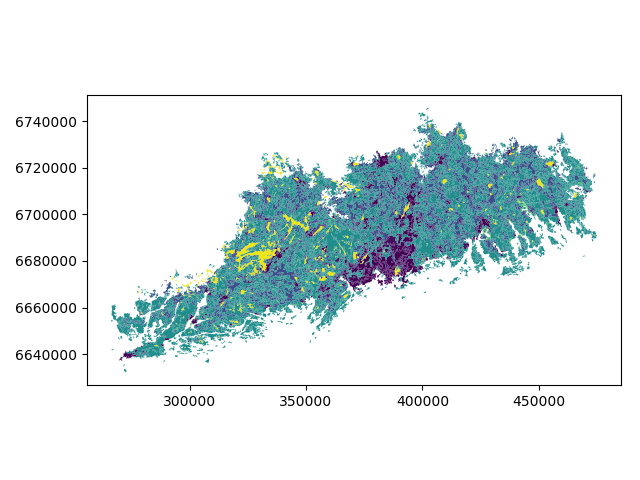Let’s see what kind of values we have in ‘Level3Eng’ column.

In : list(data['Level3Eng'].unique())
Out:
['Discontinuous urban fabric',
'Transitional woodland/shrub',
'Non-irrigated arable land',
'Fruit trees and berry plantations',
'Pastures',
'Land principally occupied by agriculture, with significant areas of natural vegetation',
'Bare rock',
'Inland marshes',
'Peatbogs',
'Salt marshes',
'Water courses',
'Water bodies',
'Sea and ocean',
'Industrial or commercial units',
'Road and rail networks and associated land',
'Port areas',
'Airports',
'Mineral extraction sites',
'Dump sites',
'Coniferous forest',
'Construction sites',
'Green urban areas',
'Sport and leisure facilities',
'Mixed forest']


Okey we have plenty of different kind of land covers in our data. Let’s select only lakes from our data. Selecting specific rows from a DataFrame based on some value(s) is easy to do in Pandas / Geopandas using a specific indexer called .ix[], read more from here..

# Select lakes (i.e. 'waterbodies' in the data) and make a proper copy out of our data
In : lakes = data.ix[data['Level3Eng'] == 'Water bodies'].copy()

Out:
Level1     Level1Eng  Level2      Level2Eng  Level3     Level3Eng  \
1388       5  Water bodies      51  Inland waters     512  Water bodies
1389       5  Water bodies      51  Inland waters     512  Water bodies

Luokka3                                           geometry
1388     512  POLYGON ((298388.189 6642944.189999999, 298364...
1389     512  POLYGON ((286629.2579999999 6643429.219000001,...


## Calculations in DataFrames¶

Okey now we have our lakes dataset ready. The aim was to classify those lakes into small and big lakes based on the average size of all lakes in our study area. Thus, we need to calculate the average size of our lakes.

Let’s check the coordinate system.

# Check coordinate system information
In : data.crs
Out: {'ellps': 'GRS80', 'no_defs': True, 'proj': 'utm', 'units': 'm', 'zone': 35}


Okey we can see that the units are in meters and we have a UTM projection.

Let’s calculate first the are of our lakes.

# Calculate the area of lakes
In : lakes['area'] = lakes.area

# What do we have?
Out:
1388    268310.708164
1389    917661.921348
Name: area, dtype: float64


Notice that the values are now in square meters.. Let’s change those into square kilometers so they are easier to read. Doing calculations in Pandas / Geopandas are easy to do:

In : lakes['area_km2'] = lakes['area'] / 1000000

# What is the mean size of our lakes?
In : l_mean_size = lakes['area_km2'].mean()

In : l_mean_size
Out: 1.5828513727796711


Okey so the size of our lakes seem to be approximately 1.58 square kilometers.

Note

It is also easy to calculate e.g. sum or difference between two or more layers (plus all other mathematical operations), e.g.:

# Sum two columns
data['sum_of_columns'] = data['col_1'] + data['col_2']

# Calculate the difference of three columns
data['difference'] = data['some_column'] - data['col_1'] + data['col_2']


## Classifying data¶

### Creating a custom classifier¶

Let’s create a function where we classify the geometries into two classes based on a given threshold -parameter. If the area of a polygon is lower than the threshold value (average size of the lake), the output column will get a value 0, if it is larger, it will get a value 1. This kind of classification is often called a binary classification.

First we need to create a function for our classification task. This function takes a single row of the GeoDataFrame as input, plus few other parameters that we can use.

def binaryClassifier(row, source_col, output_col, threshold):
# If area of input geometry is lower that the threshold value
if row[source_col] < threshold:
# Update the output column with value 0
row[output_col] = 0
# If area of input geometry is higher than the threshold value update with value 1
else:
row[output_col] = 1
# Return the updated row
return row


Let’s create an empty column for our classification

In : lakes['small_big'] = None


We can use our custom function by using a Pandas / Geopandas function called .apply(). Thus, let’s apply our function and do the classification.

In : lakes = lakes.apply(binaryClassifier, source_col='area_km2', output_col='small_big', threshold=l_mean_size, axis=1)


Let’s plot these lakes and see how they look like.

In : lakes.plot(column='small_big', linewidth=0.05, cmap="seismic")
Out: <matplotlib.axes._subplots.AxesSubplot at 0x2ce1e1cd278>

In : plt.tight_layout()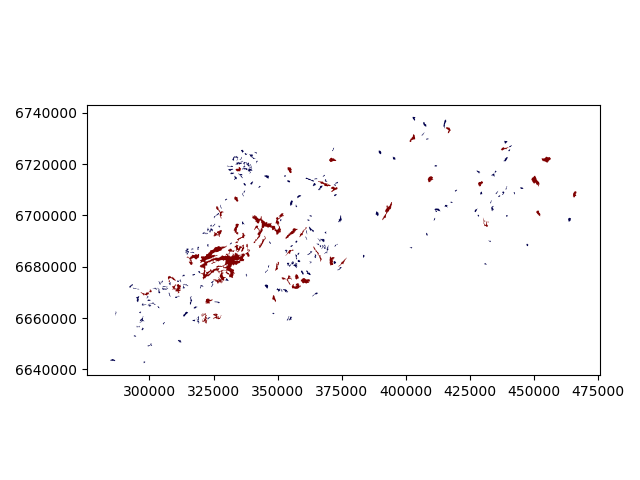Okey so it looks like they are correctly classified, good. As a final step let’s save the lakes as a file to disk.

In : outfp_lakes = r"/home/geo/lakes.shp"
In : lakes.to_file(outfp_lakes)


Note

There is also a way of doing this without a function but with the previous example might be easier to understand how the function works. Doing more complicated set of criteria should definitely be done in a function as it is much more human readable.

Let’s give a value 0 for small lakes and value 1 for big lakes by using an alternative technique:

lakes['small_big_alt'] = None
lakes.loc[lakes['area_km2'] < l_mean_size, 'small_big_alt'] = 0
lakes.loc[lakes['area_km2'] >= l_mean_size, 'small_big_alt'] = 1


### Multicriteria data classification¶

It also possible to do classifiers with multiple criteria easily in Pandas/Geopandas by extending the example that we started earlier. Now we will modify our binaryClassifier function a bit so that it classifies the data based on two columns.

Let’s call it customClassifier2 as it takes into account two criteria:

def customClassifier2(row, src_col1, src_col2, threshold1, threshold2, output_col):
# 1. If the value in src_col1 is LOWER than the threshold1 value
# 2. AND the value in src_col2 is HIGHER than the threshold2 value, give value 1, otherwise give 0
if row[src_col1] < threshold1 and row[src_col2] > threshold2:
# Update the output column with value 0
row[output_col] = 1
# If area of input geometry is higher than the threshold value update with value 1
else:
row[output_col] = 0

# Return the updated row
return row


Okey, now we have our classifier ready, let’s use it to our data.

First, we need to read our Travel Time data from Helsinki into memory from the GeoJSON file that we prepared earlier with overlay analysis.

fp = r"/home/geo/TravelTimes_to_5975375_RailwayStation_Helsinki.geojson"

# Read the GeoJSON file similarly as Shapefile

# Let's see what we have


Okey we have plenty of different variables (see from here the description for all attributes) but what we are interested in are columns called pt_r_tt which is telling the time in minutes that it takes to reach city center from different parts of the city, and walk_d that tells the network distance by roads to reach city center from different parts of the city (almost equal to Euclidian distance).

The NoData values are presented with value -1. Thus we need to remove those first.

In : acc = acc.ix[acc['pt_r_tt'] >=0]


Let’s plot it and see how our data looks like.

In : import matplotlib.pyplot as plt

# Plot using 9 classes and classify the values using "Fisher Jenks" classification
In : acc.plot(column="pt_r_tt", scheme="Fisher_Jenks", k=9, cmap="RdYlBu", linewidth=0);

# Use tight layour
In : plt.tight_layout()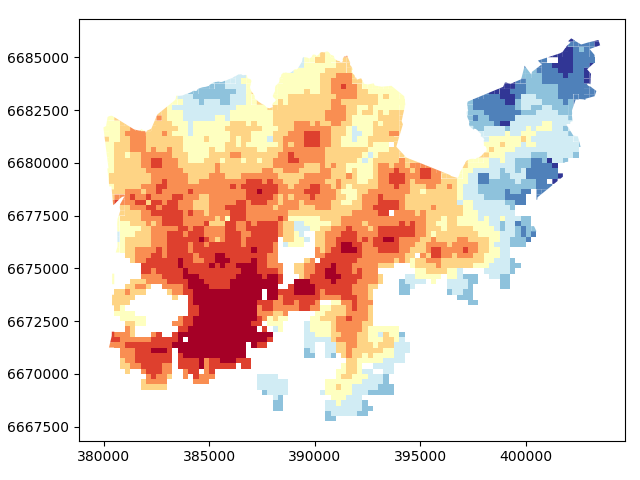Okey so from this figure we can see that the travel times are lower in the south where the city center is located but there are some areas of “good” accessibility also in some other areas (where the color is red).

Let’s also make a plot about walking distances

In : acc.plot(column="walk_d", scheme="Fisher_Jenks", k=9, cmap="RdYlBu", linewidth=0);

# Use tight layour
In : plt.tight_layout();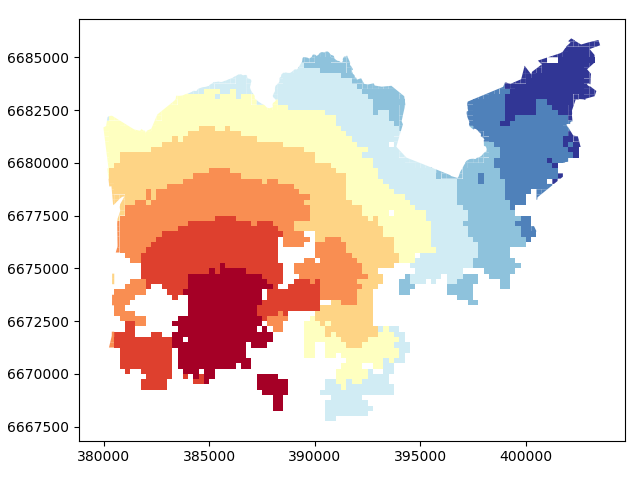Okey, from here we can see that the walking distances (along road network) reminds more or less Euclidian distances.

Let’s finally do our classification based on two criteria and find out grid cells where the travel time is lower or equal to 20 minutes but they are further away than 4 km (4000 meters) from city center.

Let’s create an empty column for our classification results called “Suitable_area”.

In : acc["Suitable_area"] = None


Now we are ready to apply our custom classifier to our data with our own criteria.

In : acc = acc.apply(customClassifier2, src_col1='pt_r_tt', src_col2='walk_d', threshold1=20, threshold2=4000, output_col="Suitable_area", axis=1)


Let’s see what we got.

In : acc.head()
Out:
car_m_d  car_m_t  car_r_d  car_r_t  from_id  pt_m_d  pt_m_t  pt_m_tt  \
0    15981       36    15988       41  6002702   14698      65       73
1    16190       34    16197       39  6002701   14661      64       73
2    15727       33    15733       37  6001132   14256      59       69
3    15975       33    15982       37  6001131   14512      62       73
4    16136       35    16143       40  6001138   14730      65       73

pt_r_d  pt_r_t      ...         to_id  walk_d  walk_t    GML_ID   NAMEFIN  \
0   14698      61      ...       5975375   14456     207  27517366  Helsinki
1   14661      60      ...       5975375   14419     206  27517366  Helsinki
2   14256      55      ...       5975375   14014     200  27517366  Helsinki
3   14512      58      ...       5975375   14270     204  27517366  Helsinki
4   14730      61      ...       5975375   14212     203  27517366  Helsinki

NAMESWE NATCODE          area  \
0  Helsingfors     091  62499.999976
1  Helsingfors     091  62499.999977
2  Helsingfors     091  62499.999977
3  Helsingfors     091  62499.999976
4  Helsingfors     091  62499.999977

geometry Suitable_area
0  POLYGON ((391000.0001349226 6667750.00004299, ...             0
1  POLYGON ((390750.0001349644 6668000.000042951,...             0
2  POLYGON ((391000.0001349143 6668000.000042943,...             0
3  POLYGON ((390750.0001349644 6668000.000042951,...             0
4  POLYGON ((392500.0001346234 6668000.000042901,...             0

[5 rows x 21 columns]


Okey we have new values in Suitable_area .column.

How many Polygons are suitable for us? Let’s find out by using a Pandas function called value_counts() that return the count of different values in our column.

In : acc['Suitable_area'].value_counts()
Out:
0    3808
1       9
Name: Suitable_area, dtype: int64


Okey so there seems to be nine suitable locations for us where we can try to find an appartment to buy Let’s see where they are located.

# Plot
In : acc.plot(column="Suitable_area", linewidth=0);

# Use tight layour
In : plt.tight_layout();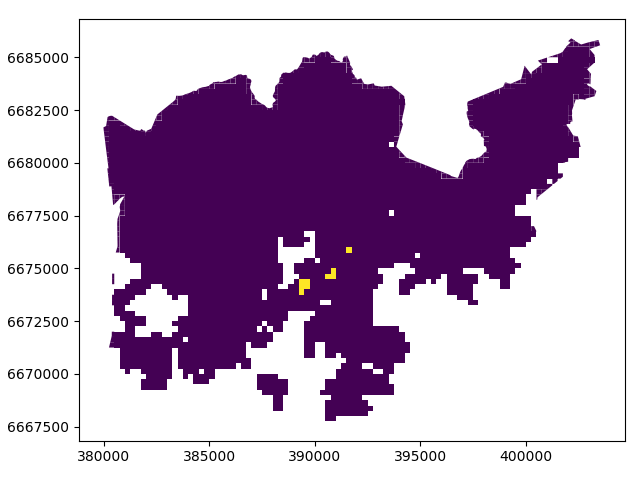A-haa, okey so we can see that suitable places for us with our criteria seem to be located in the eastern part from the city center. Actually, those locations are along the metro line which makes them good locations in terms of travel time to city center since metro is really fast travel mode.

Todo

Try to change your classification criteria and see how your results change! What places would be suitable for you to buy an apartment in Helsinki region? You can also change the travel mode and see how they change the results.

### Classification based on common classifiers¶

Pysal -module is an extensive Python library including various functions and tools to do spatial data analysis. It also includes all of the most common data classifiers that are used commonly e.g. when visualizing data. Available map classifiers in pysal -module are (see here for more details):

• Box_Plot
• Equal_Interval
• Fisher_Jenks
• Fisher_Jenks_Sampled
• Jenks_Caspall
• Jenks_Caspall_Forced
• Jenks_Caspall_Sampled
• Max_P_Classifier
• Maximum_Breaks
• Natural_Breaks
• Quantiles
• Percentiles
• Std_Mean
• User_Defined

Let’s apply one of those classifiers into our data and classify the travel times by public transport into 9 classes.

In : import pysal as ps

# Define the number of classes
In : n_classes = 9


The classifier needs to be initialized first with make() function that takes the number of desired classes as input parameter.

# Create a Natural Breaks classifier
In : classifier = ps.Natural_Breaks.make(k=n_classes)


Now we can apply that classifier into our data quite similarly as in our previous examples.

# Classify the data
In : classifications = acc[['pt_r_tt']].apply(classifier)

# Let's see what we have
Out:
pt_r_tt
0        7
1        7
2        6
3        7
4        7


Okey, so we have a DataFrame where our input column was classified into 9 different classes (numbers 1-9) based on Natural Breaks classification.

Now we want to join that reclassification into our original data but let’s first rename the column so that we recognize it later on.

# Rename the column so that we know that it was classified with natural breaks
In : classifications.columns = ['nb_pt_r_tt']

# Join with our original data (here index is the key
In : acc = acc.join(classifications)

# Let's see how our data looks like
Out:
car_m_d  car_m_t  car_r_d  car_r_t  from_id  pt_m_d  pt_m_t  pt_m_tt  \
0    15981       36    15988       41  6002702   14698      65       73
1    16190       34    16197       39  6002701   14661      64       73
2    15727       33    15733       37  6001132   14256      59       69
3    15975       33    15982       37  6001131   14512      62       73
4    16136       35    16143       40  6001138   14730      65       73

pt_r_d  pt_r_t    ...      walk_d  walk_t    GML_ID   NAMEFIN      NAMESWE  \
0   14698      61    ...       14456     207  27517366  Helsinki  Helsingfors
1   14661      60    ...       14419     206  27517366  Helsinki  Helsingfors
2   14256      55    ...       14014     200  27517366  Helsinki  Helsingfors
3   14512      58    ...       14270     204  27517366  Helsinki  Helsingfors
4   14730      61    ...       14212     203  27517366  Helsinki  Helsingfors

NATCODE          area                                           geometry  \
0     091  62499.999976  POLYGON ((391000.0001349226 6667750.00004299, ...
1     091  62499.999977  POLYGON ((390750.0001349644 6668000.000042951,...
2     091  62499.999977  POLYGON ((391000.0001349143 6668000.000042943,...
3     091  62499.999976  POLYGON ((390750.0001349644 6668000.000042951,...
4     091  62499.999977  POLYGON ((392500.0001346234 6668000.000042901,...

Suitable_area nb_pt_r_tt
0              0          7
1              0          7
2              0          6
3              0          7
4              0          7

[5 rows x 22 columns]


Great, now we have those values in our accessibility GeoDataFrame. Let’s visualize the results and see how they look.

# Plot
In : acc.plot(column="nb_pt_r_tt", linewidth=0, legend=True);

# Use tight layour
In : plt.tight_layout()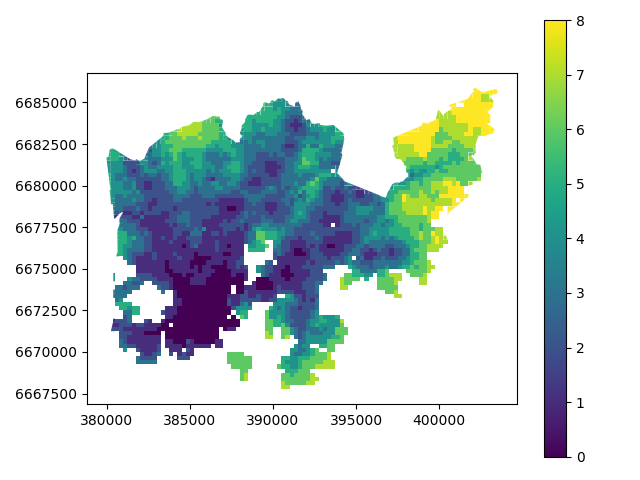And here we go, now we have a map where we have used one of the common classifiers to classify our data into 9 classes.